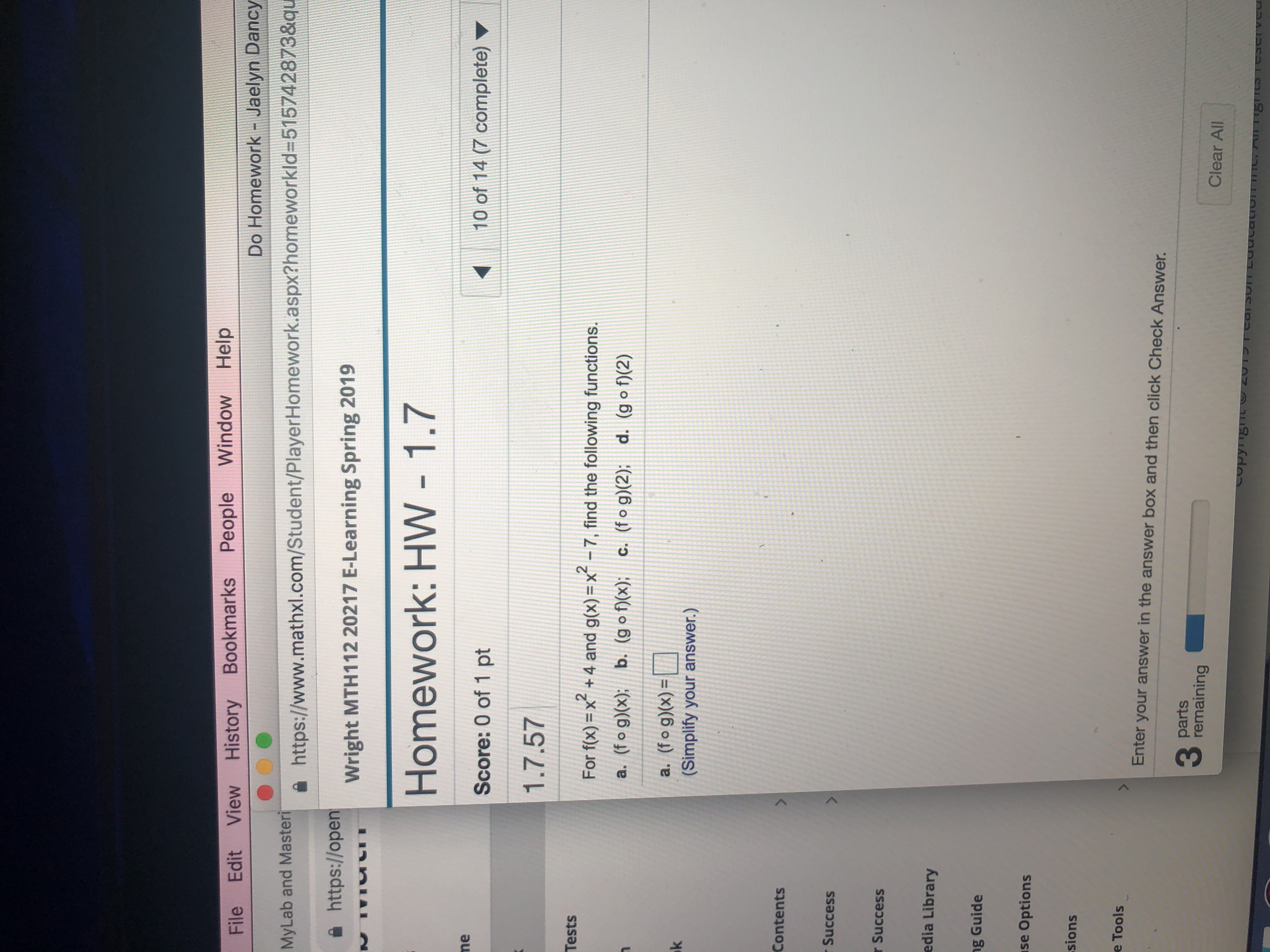# File Edit View History Bookmarks People Window HelpDo Homework -Jaelyn DancyMyLab and Masterihttps://www.mathxl.com/Student/PlayerHomework.aspx?homeworkid=515742873&que https://openWright MTH112 20217 E-Learning Spring 2019Homework: HW 1.7neScore: 0 of 1 pt10 of 14 (7 complete)1.7.57TestsFor fo -x2 +4 and g()-X2 -7, find the following functions.a. (f o g)) b. (g o): c. (fog)(2) d. (g o(2)a. (fog)(x)=(Simplify your answer.)ContentsSuccessSuccessedia Libraryg Guidese Optionssionse ToolsEnter your answer in the answer box and then click Check AnswerpartsremainingClear All

Questionhelp_outlineImage TranscriptioncloseFile Edit View History Bookmarks People Window Help Do Homework -Jaelyn Dancy MyLab and Masteri https://www.mathxl.com/Student/PlayerHomework.aspx?homeworkid=515742873&qu e https://open Wright MTH112 20217 E-Learning Spring 2019 Homework: HW 1.7 ne Score: 0 of 1 pt 10 of 14 (7 complete) 1.7.57 Tests For fo -x2 +4 and g()-X2 -7, find the following functions. a. (f o g)) b. (g o): c. (fog)(2) d. (g o(2) a. (fog)(x)= (Simplify your answer.) Contents Success Success edia Library g Guide se Options sions e Tools Enter your answer in the answer box and then click Check Answer parts remaining Clear All fullscreen
check_circle

Step 1

Note: Since this question is a multi-part question. As per the subpart rule, we will be providing answers for first three sub parts.

Step 2

We have been given two function f(x)=x^2+4 and g(x)=x^2-7 and we need to compose these functions to find the stated composite functions.

Step 3

Part (a)

In order to find (fog)(x) we need to use the defintion of composition functions (fog)(x) = f(g(x)) and we ne...

### Want to see the full answer?

See Solution

#### Want to see this answer and more?

Solutions are written by subject experts who are available 24/7. Questions are typically answered within 1 hour.*

See Solution
*Response times may vary by subject and question.
Tagged in

### Calculus